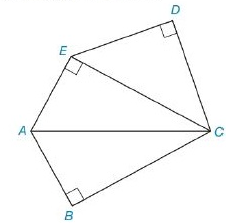Chapter 8.2, Problem 29EElementary Geometry For College St...

7th Edition
Alexander + 2 others
ISBN: 9781337614085

Solutions

Chapter
SectionElementary Geometry For College St...

7th Edition
Alexander + 2 others
ISBN: 9781337614085
Textbook Problem

In Exercises 27 to 30, find the area of the figure shown. Given: Pentagon ABCDEwith D C - ≅ D E - A E = A B = 5 B C = 12 Find: A A B C D ETo determine

To Find:

Area of the pentagon provided.

Explanation

Formula Used:

Split the pentagon into three triangles and find the corresponding areas and then sum it up.

Pythagorean theorem for the right angle triangle ABC for the hypotenuse AC,

AC2=AB2+BC2.

Area of triangle =12bh.

Calculation:

Let the vertices of the provided pentagon be A,B,C,D and E.

Let’s divide the pentagon into three triangles. That is, CDE, ACE and ABC.

It is given that mCDE=mCBA=mCEA=90°,  DC-DE-, AE=AB=5 and BC=12.

Consider the triangles ACE and ABC,

Here AE=AB=5 and both the triangles have common base. They are similar triangles. Therefore, the side EC must be equal to BC. That is, EC=BC. As the triangles have same side lengths, their areas will also be same.

Area of ΔABC=12bh=12×5×12=30

Still sussing out bartleby?

Check out a sample textbook solution.

See a sample solution

The Solution to Your Study Problems

Bartleby provides explanations to thousands of textbook problems written by our experts, many with advanced degrees!

Get Started

Find more solutions based on key concepts